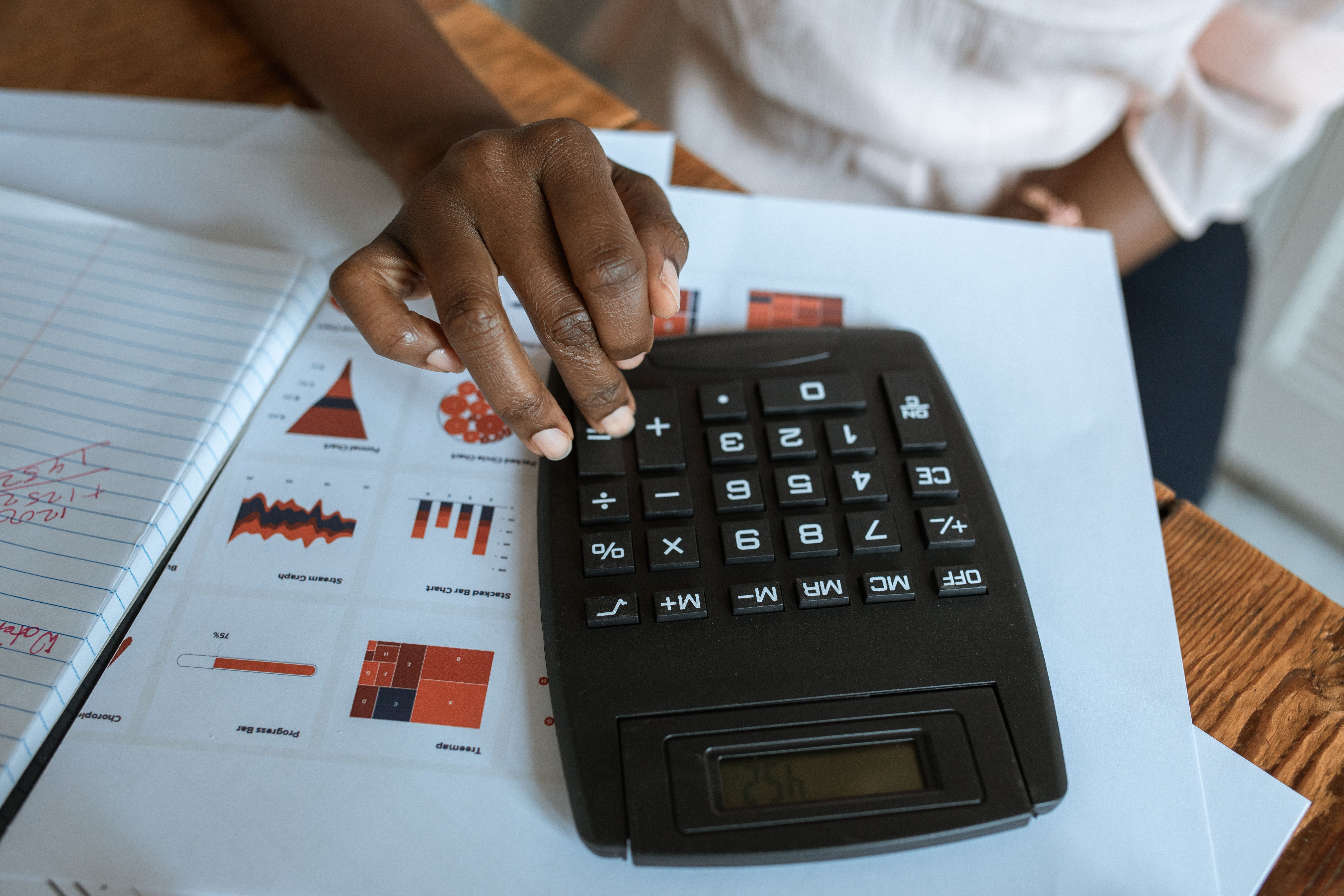# Why is physics so much math?

Why Physics is so much Math?

# Why Physics is so much Math?## Introduction

Physics is a fundamental branch of science that relies heavily on mathematics to solve problems and make predictions. Many people wonder why physics requires so much math, and this article aims to answer that question.

## FAQ about Physics and Math

### Why is math important in physics?

Math plays a critical role in scientific calculation in the field of physics. Physics carries significant crossover with several mathematical fields, including algebra for basic physics and calculus for advanced physics. Math is important in physics because it allows scientists to describe physical phenomena using equations that can be used to predict future events or to understand how things work.

### What math skills are necessary for physics?

To be successful in physics, one must have a strong foundation in algebra and trigonometry. Additionally, calculus is essential for advanced physics concepts such as electromagnetism and the theory of relativity. Differential equations are also important for solving complex physics problems.

### Can you study physics without being good at math?

It is possible to study physics without being a math whiz, but it will be challenging. It would be best to have a good understanding of math concepts before diving into physics classes. One option for those who struggle with math but want to study physics is to get help from a tutor or to work through introductory math courses before diving into physics.

### Why do physicists love math?

Physicists love math because it allows them to describe physical phenomena in a concise and precise way. Math provides physicists with tools to model reality, make predictions, and test hypotheses. It is also the language of physics, allowing physicists from around the world to share ideas and collaborate on research projects.

### How can math be used to solve physics problems?

Math can be used to solve physics problems by setting up equations that describe the physical phenomenon being studied. For example, to calculate the velocity of an object in motion, one would use the equation v = d/t, where v is the velocity, d is the distance traveled, and t is the time it took to travel that distance. By plugging in the appropriate values, one can determine the velocity.

## The Relationship Between Physics and MathPhysics and math have a fascinating relationship. Although they are distinct fields, they are deeply interconnected, and advancements in one often lead to improvements in the other. Math provides the language and tools for describing complex physical phenomena, making it an essential tool for physicists.

Physics also helps to drive advances in math. When physicists encounter new and complex phenomena that cannot be described by existing math, they often work with mathematicians to develop new mathematical tools and concepts to solve the problem. This collaboration leads to innovation in both fields.

## Advanced Physics Concepts and MathAdvanced physics concepts often require advanced math skills to understand and solve problems. Concepts like relativity and quantum mechanics require knowledge of calculus, linear algebra, and differential equations. These mathematical tools are essential for physicists who want to describe and predict the behavior of the universe at the most fundamental levels.